Maths Practice Questions

FREE quiz to give you a flavour of the types of questions covered: MATHS SAMPLE QUIZ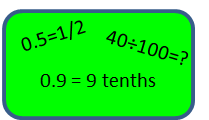Number Notation

General number work including decimal place values, fractions and multiplying by 10, 100 and 1000.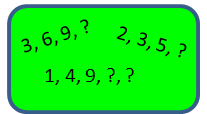Patterns, Relationships & Sequences

Questions including sequences, multiples, factors, prime, square and cube numbers.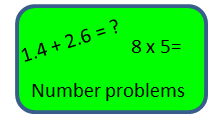Operations & Their Applications

Number questions testing the +, -, / and x functions, including the application to decimals.Money

Working with money e.g. 50% sale, and how to interpret a calculator display in relation to money.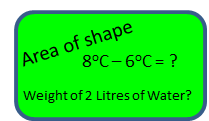Measures

Testing on all things related to length, weight, volume, time area and temperature.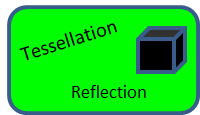Shape & Space

Questions focused on shapes (squares, rectangles, circles, triangles, hexagons, pentagons) and includes reflection & tessellation.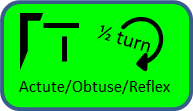Position, Movement & Direction

Checking understanding on 1/4 turns, 1/2 turns, clockwise and anticlockwise.  Also testing on the terms vertical, horizontal, perpendicular, parallel, acute, obtuse and reflex.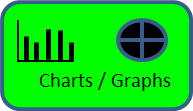Interpret Data

Working with questions that require understanding of graphs, tables, Venn, decision tree, Carroll diagrams, pictograms, block graphs, bar charts and bar-line graphs.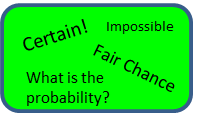Probability

Questions to test understanding of certain, uncertain, likely, unlikely, impossible and fair.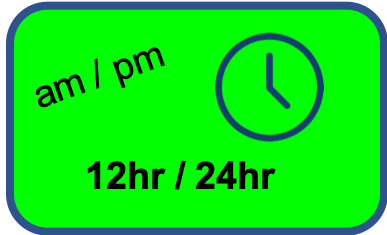Time

Exploring all elements of time including am/pm and the 12hr and 24hr clock.